NCERT Solutions for Class 8 Maths Chapter 6 Squares and Square Roots Ex 6.3 are part of NCERT Solutions for Class 8 Maths. Here we have given NCERT Solutions for Class 8 Maths Chapter 6 Squares and Square Roots Ex 6.3.

 Board CBSE Textbook NCERT Class Class 8 Subject Maths Chapter Chapter 6 Chapter Name Squares and Square Roots Exercise Ex 6.3 Number of Questions Solved 10 Category NCERT Solutions

## NCERT Solutions for Class 8 Maths Chapter 6 Squares and Square Roots Ex 6.3

Question 1.
What could be the possible ‘one’s’ digits of the square root of each of the following numbers ?
(i) 9801
(ii) 99856
(iii) 998001
(iv) 657666025.
Solution.
(i) 9801
∵ 1 x 1 = 1 and 9 x 9 = 81
∵ The possible one’s digit of the square root of the number 9801 could be 1 or 9.

(ii) 99856
∵ 4 x 4 = 16 and 6 x 6 = 36
∵ The possible one’s digit of the square root of the number 99856 could be 4 or 6.

(iii) 998001
∵ 1×1 = 1 and 9 x 9 = 81
∵ The possible one’s digit of the square root of the number 998001 could be 1 or 9.

(iv) 657666025
∵ 5 x 5 = 25
∵ The possible one’s digit of the square root of the number 657666025 could be 5.

Question 2.
Without doing any calculation, find the numbers which are surely not perfect squares.
(i) 153
(ii) 257
(iii) 408
Solution.
(i) 153
The number 153 is surely not a perfect square because it ends in 3 whereas the square numbers end with 0, 1, 4, 5, 6 or 9.

(ii) 257
The number 257 is surely not a perfect square because it ends in 7 whereas the square numbers end with 0, 1, 4, 5, 6 or 9.

(iii) 408
The number 408 is surely not a perfect square because it ends in 8 whereas the square numbers end with 0, 1, 4, 5, 6 or 9.

(iv) 441
The number may be a perfect square as the square numbers end wTith 0, 1, 4, 5, 6 or 9.

Question 3.
Find the square roots of 100 and 169 by the method of repeated subtraction.
Solution.
(i) 100

• 100 – 1 = 99
• 99 – 3 = 96
• 96 – 5 = 91
• 91 – 7 = 84
• 84 – 9 = 75
• 75 – 11 = 64
• 64 – 13 = 51
• 51 – 15 = 36
• 36 – 17 = 19
• 19 – 19 = 0

Since from 100, we subtracted successive odd numbers starting from 1 and obtained 0 at the 10th step, therefore,
$$\sqrt { 100 } =10$$

(ii) 169

• 169 – 1 = 168
• 168 – 3 = 165
• 165 – 5 = 160
• 160 – 7 = 153
• 153 – 9 = 144
• 144-11 = 133
• 133 – 13 = 120
• 120 – 15 = 105
• 105 – 17 = 88
• 88 – 19 = 69
• 69 – 21 = 48
• 48 – 23 = 25
• 25 – 25 = 0

Since rom 169, we subtracted successive odd numbers starting from 1 and obtained 0 at the 13th step, therefore,
$$\sqrt { 169 } =13$$

Question 4.
Find the square roots of the following numbers by the Prime Factorisation Method:
(i) 729
(ii) 400
(iii) 1764
(iv) 4095
(v) 7744
(vi) 9604
(vii) 5929
(viii) 9216
(ix) 529
(x) 8100
Solution.
(i) 729
The prime factorisation of 729 is
729 = 3 x 3 x 3 x 3 x 3 x 3.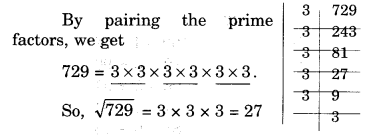(ii) 400
The prime factorisation of 400 is
400 = 2 x 2 x 2 x 2 x 5 x 5.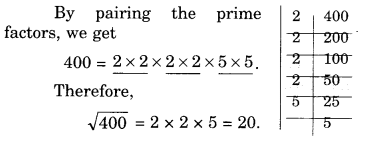(iii) 1764(iv) 4096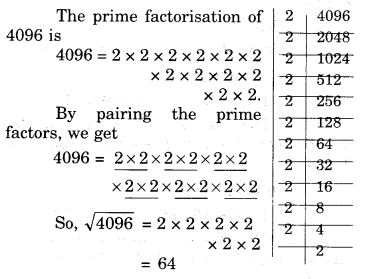(v) 7744(vi) 9604(vii) 5929(viii) 9216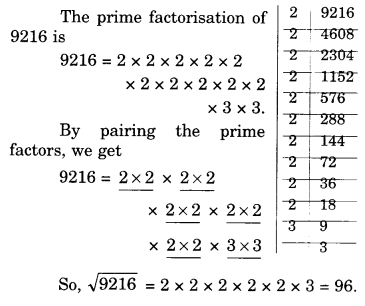(ix) 529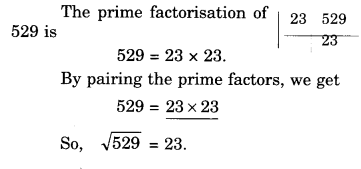(x) 8100Question 5.
For each of the following numbers, find the smallest whole number by which it should be multiplied so as to get a perfect square number. Also find the square root of the square number so obtained.
(i) 252
(ii) 180
(iii) 1008
(iv) 2028
(v) 1458
(vi) 768
Solution.
(i) 252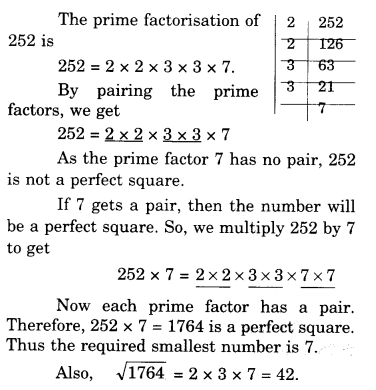(ii) 180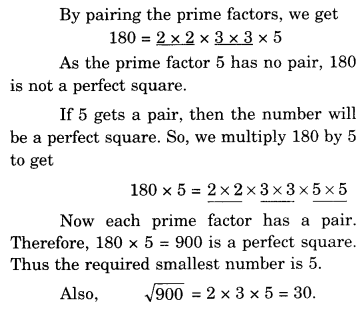(iii) 1008(iv) 2028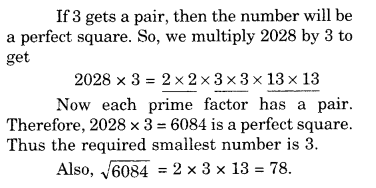(v) 1458(vi) 768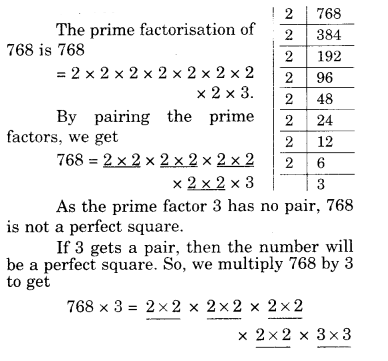Question 6.
For each of the following numbers, find the smallest whole number by which it should be divided so as to get a perfect square. Also find the square root of the square number so obtained.
(i) 252
(ii) 2925
(iii) 396
(iv) 2645
(v) 2800
(vi) 1620
Solution.
(i) 252(ii) 2925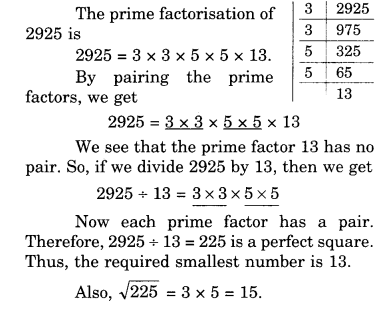(iii) 396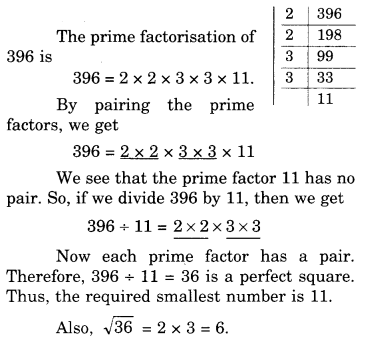(iv) 2645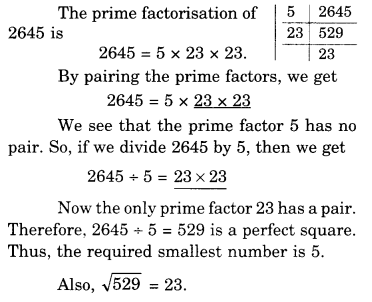(v) 2800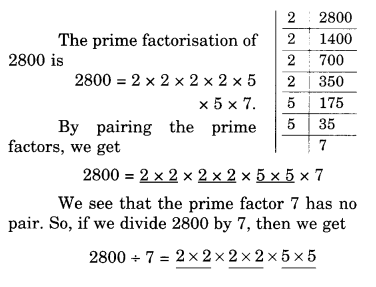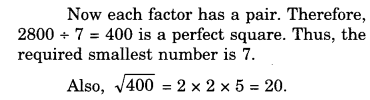(vi) 1620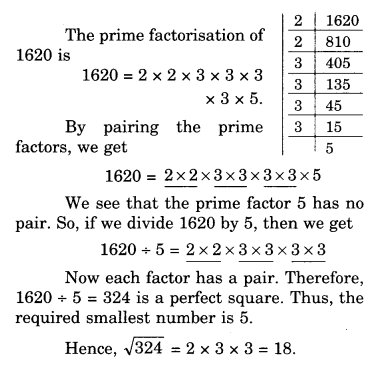Question 7.
The students of Class VIII of a school donated? 2401 in all, for Prime Minister’s National Relief Fund. Each student donated as rr’iny rupees as the number of students in the class. Find the number of students in the class.
Solution.
Let the number of students in the class be x.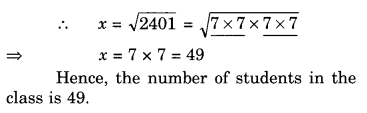Question 8.
2025 plants are to be planted in a garden in such a way that each row contains as many plants as the number of rows. Find the number of rows and the number of plants in each row.
Solution.
Let the number of rows be x.
Then, number of plants in each row = x.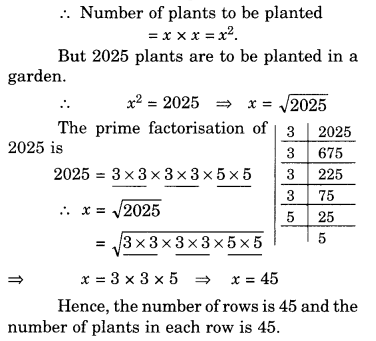Question 9.
Find the smallest square number that is divisible by each of the numbers 4, 9 and 10.
Solution.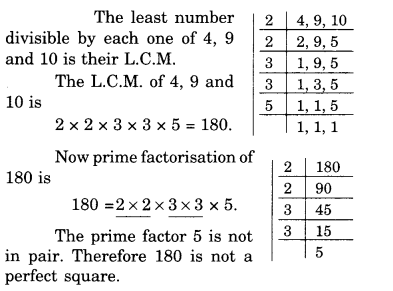In order to get a perfect square, each factor of 180 must be paired. So, we need to make pair of 5.
Therefore, 180 should be multiplied by 5.
Hence, the required smallest square number is 180 x 5 = 900.

Question 10.
Find the smallest square number that is divisible by each of the numbers 8, 15 and 20.
Solution.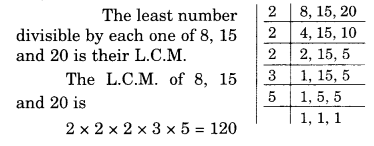We hope the NCERT Solutions for Class 8 Maths Chapter 6 Squares and Square Roots Ex 6.3 are part help you. If you have any query regarding NCERT Solutions for Class 8 Maths Chapter 6 Squares and Square Roots Ex 6.3 are part, drop a comment below and we will get back to you at the earliest.